/ EN

# How ICMIZER Makes Calculations

### Contents

ICMIZER has a reputation that it's built up deservedly over a long time as an accurate and reliable tool for finding the optimal preflop strategy using FGS, ICM, or a simple ChipEV model. Over the past few years, not a single naive error has been found in ICMIZER's calculations. Every day, hundreds of users from around the world use ICMIZER to make calculations and find optimal preflop solutions for two reasons: 1) because the calculations are very complex, and 2) because the calculation engine has been developed and improved upon for nearly 10 years. ICMIZER's calculations are used to guide the best poker players, and its results are trusted by thousands of players worldwide.

However, we regularly receive questions from beginners or users who are simply curious and sometimes skeptical about how ICMIZER makes its calculations, what formulas and data it uses, how to check the reliability of calculations, and so on. It's very difficult to answer these questions both succinctly and in detail, but we'll try to do so.

So, how does ICMIZER make calculations?

## Let's interpret calculations

"Click the ICMIZE button, and ICMIZER will calculate and generate the optimal push/fold table for the given tournament situation."

You might see this sentence in many of our articles, manuals, and tutorials, but let's take a closer look at what's being generated.

We'll analyze the result of a calculation for the first hand from our article How to learn tournament Poker Strategy with ICMIZER 3.

https://www.icmpoker.com/icmizer/#NTdyoT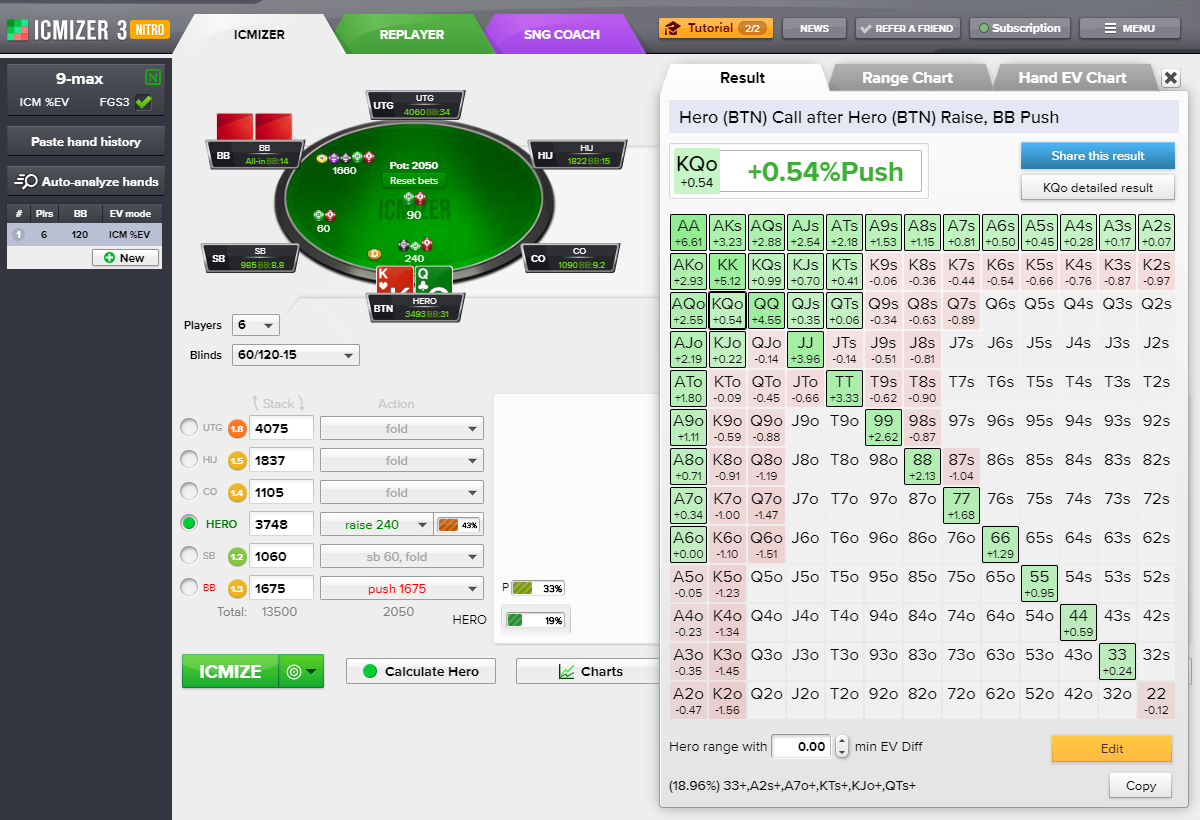The values on the pushfold table show the difference between EvPush and EvFold. Select push or call if EvCall is bigger than EvFold. Hands like these are green in the pushfold table. By and large, the principle behind the pushfold table is easy: if we see that a hand is green on the pushfold table, we push!

When selecting ICM \$EV as the calculation type, the values are displayed in dollars. For ICM %EV, the values are shown as a percentage of the tournament's total prize pool. Chip EV is the value expressed in tournament chips, and Chip BB EV is the value expressed in big blinds.

ICMIZER's calculation is complete and takes into account the blinds players place and the card removal effect. You don't need to adjust anything; ICMIZER offers a complete finished result that doesn't require any adjustments.

How to verify that?

To show what this looks like, let's build a hand from a 4-max shootout satellite, even player stacks with 1,500 chips and 50/100 blinds.

CO and BTN fold, SB push, Hero on BB.

https://www.icmpoker.com/icmizer/#sbufEs

In a cash game or shootout tournament, like this one, we can use the ChipEV model. With 50/100 blinds, the player's stack will be 1,400 chips after the fold. That means the EvFold calculation is simple: t1400.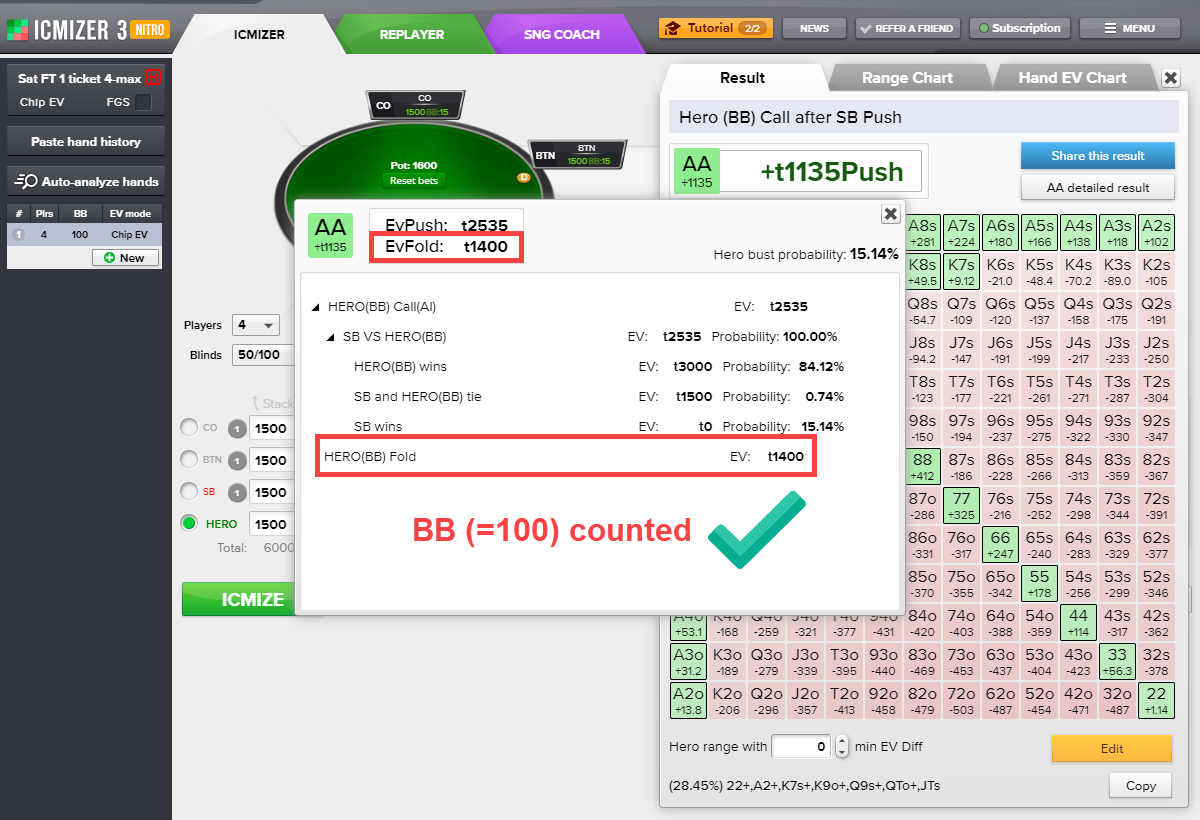If you need to calculate a tournament hand structured differently from the shootout satellite payout structure used above, use the ICM calculation model. With these conditions, each player has a 25% EV at the start of the hand. Since ICMIZER calculations take into account posted blinds, the EV for the Hero Fold will be 25% less: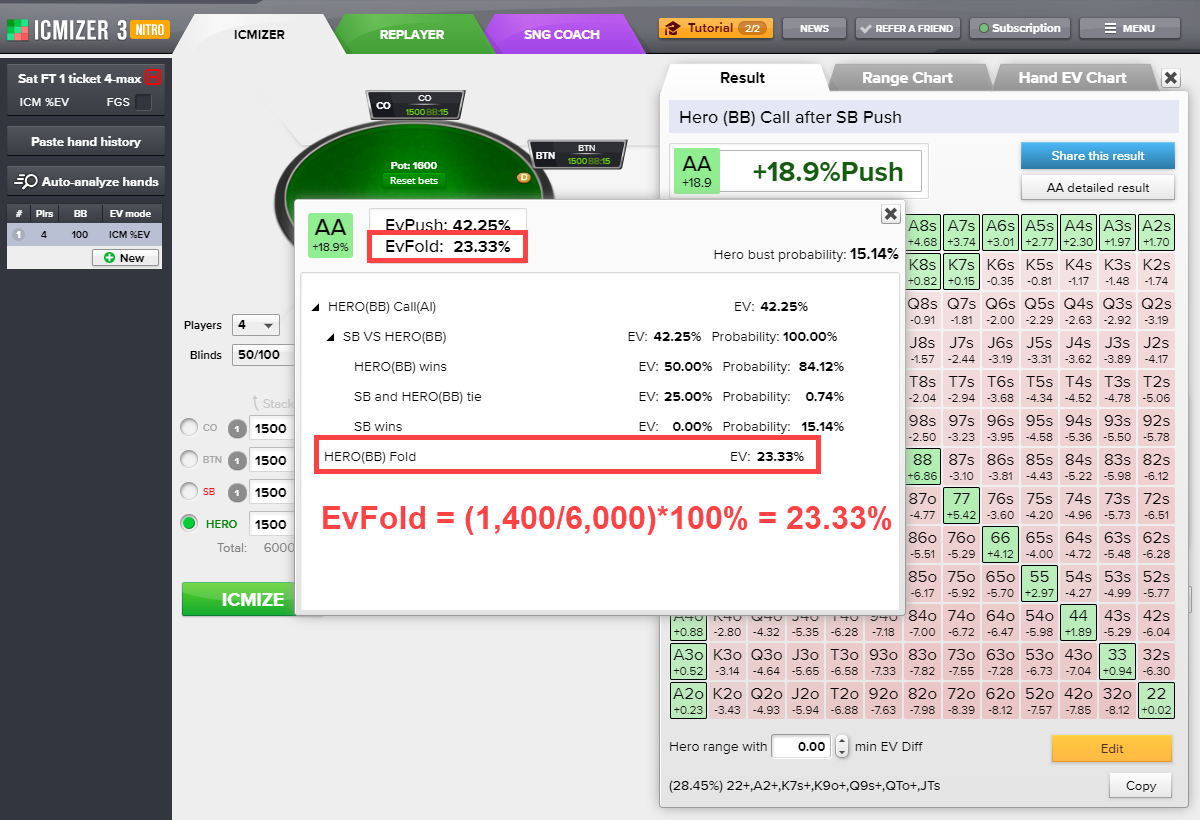## Detailed result

The break-down of the hand's calculation for each possible hand outcome is available in the detailed result window. Click 'KQo detailed result' to see details for a KQo hand's calculation.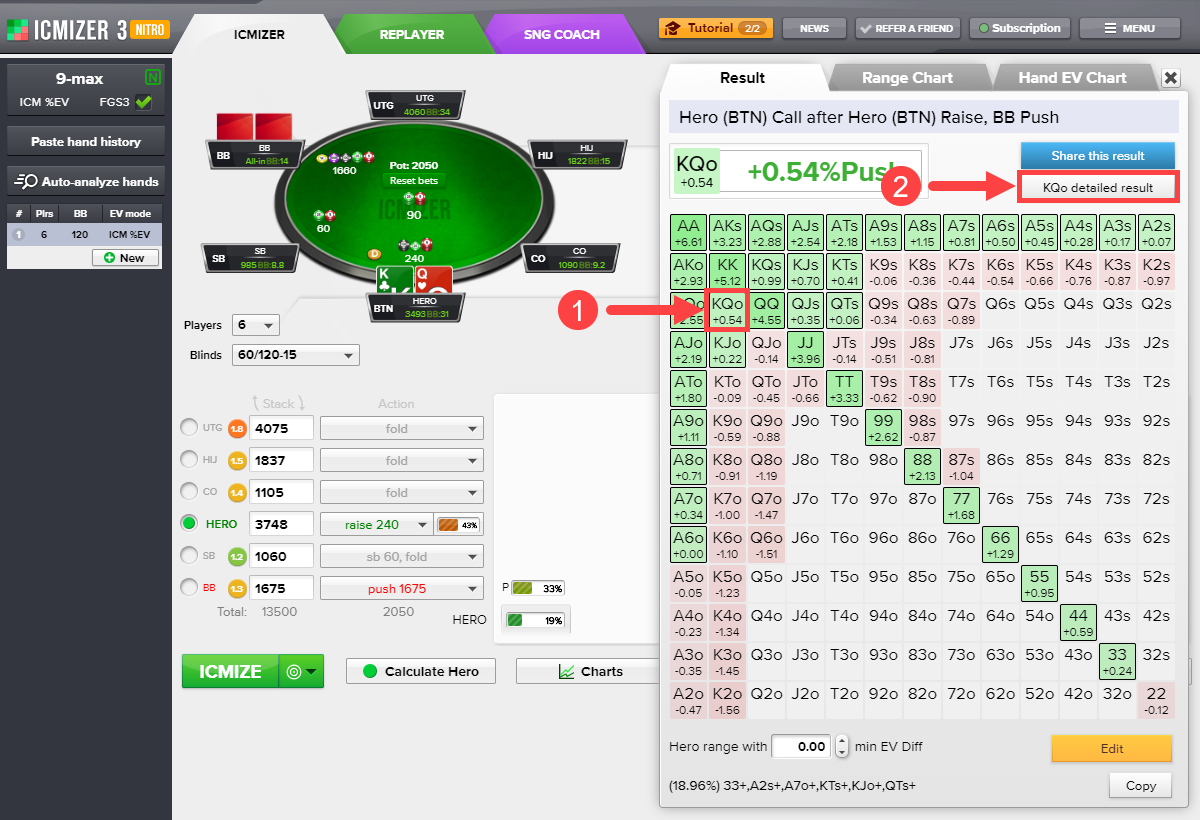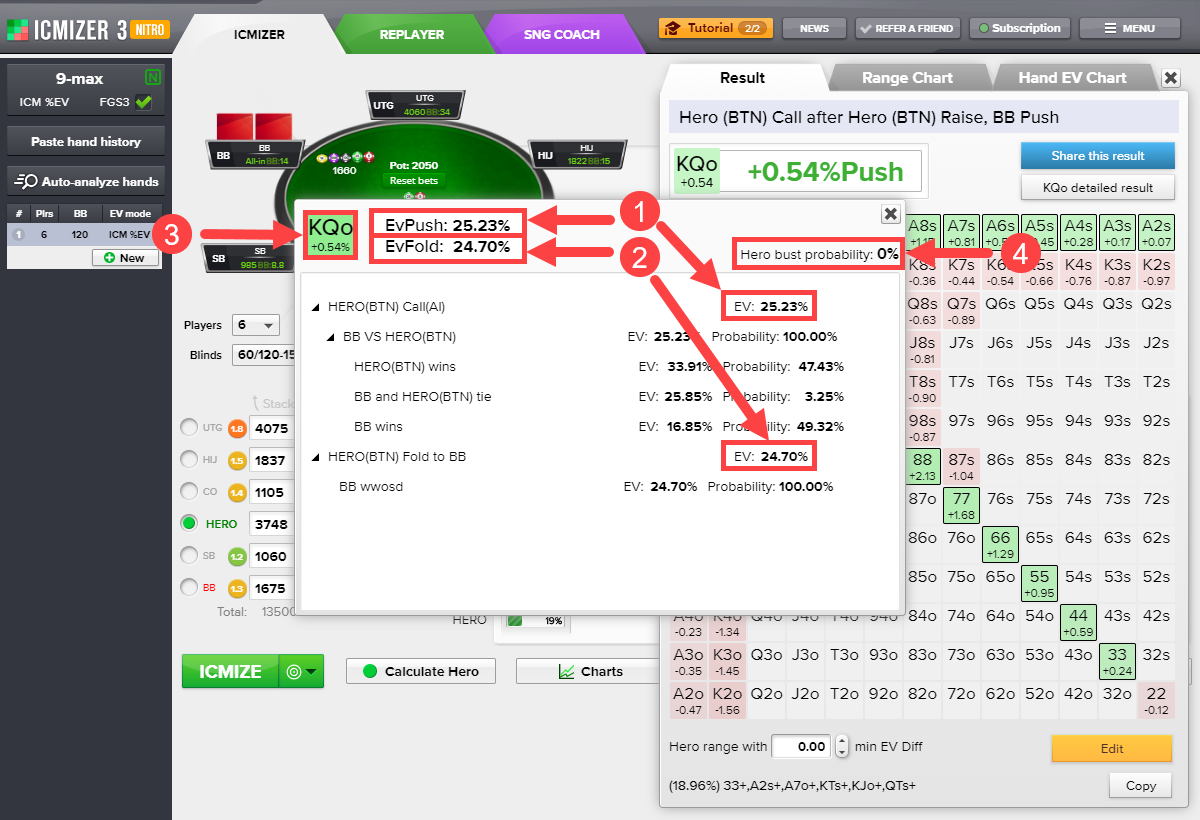In the detailed result window, you'll find:

(1) The hero's equity is expressed as a percentage of the total tournament prize pool if he goes all-in, EvPush = 25.23%. In this case, ICMIZER analyzed three possible outcomes: the hero wins (HERO(BTN) wins), the BB player wins (BB wins), and tie (BB and HERO(BTN) tie). ICMIZER also calculated the probability for each outcome.

(2) The hero's equity is expressed as a percentage of the total tournament prize pool if he folds, EvPush = 24.70%. In this case, the EvFold calculation is simple: the BB takes the whole pot.

(3) The profitability of the hero's call. The value +0.54% is obtained from the formula (EvPush – EvFold). Green means that it's more profitable to push this hand than to fold since EvPush is bigger than EvFold.

(4) The probability of the hero being busted if he's all-in. Since the hero's stack is larger than the BB's stack, the probability of being busted is 0%.

How do we get these numbers?

## How ICM calculations are made

Let's take a look at how ICM calculations are made. We'll take a simple example of calculating the expected value (EV) of open pushing AA from the button using the ICM model.

https://www.icmpoker.com/icmizer/#gEAsdF

Classic 6-max Sit'n'Go, payouts 65/35
Blinds 100/200-25, 3 players
Hero (BTN): 4,000
SB: 3,000
BB: 2,000

Preflop: Hero is on the BTN with AA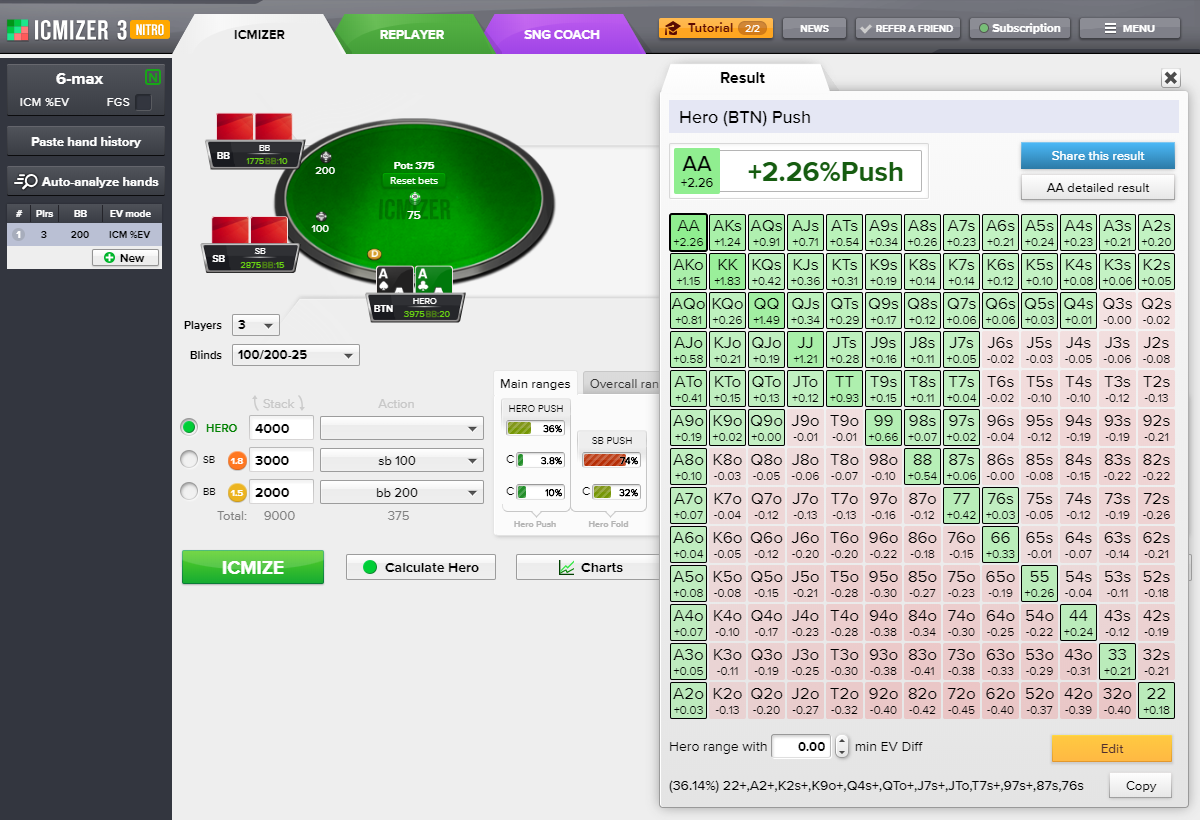1. First, we need to build a probability tree for all possible outcomes after we push. You can build it yourself or open the probability tree that's already been generated in ICMIZER.

To see the probability chart of possible outcomes in ICMIZER, open the calculation break-down by clicking 'AA detailed result'.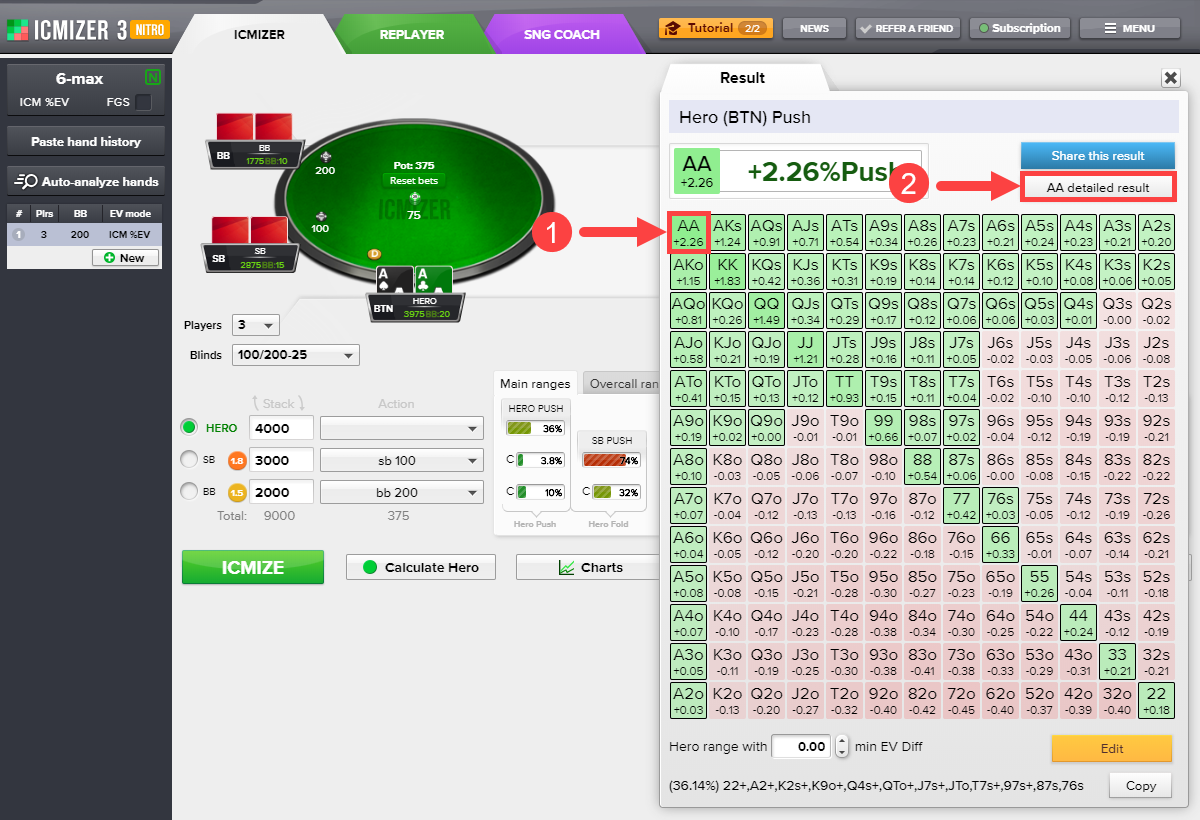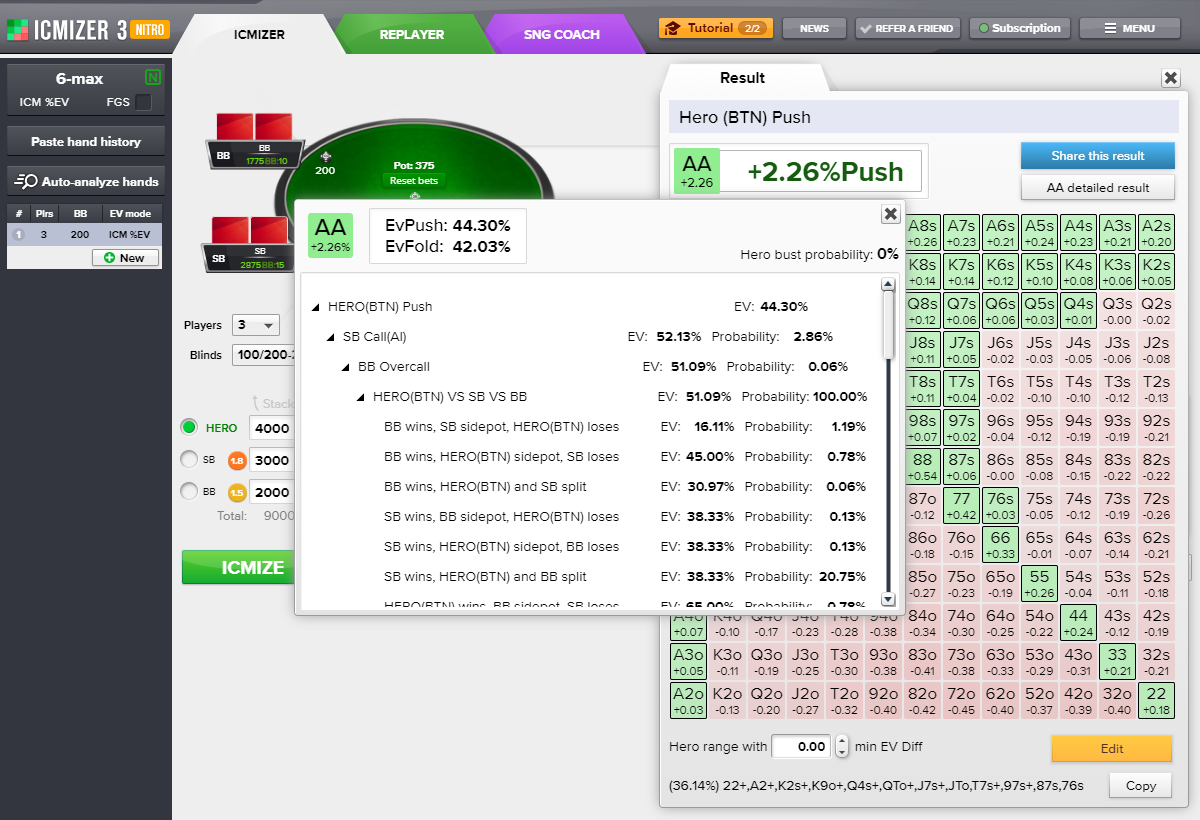Here's what the full probability tree for possible outcomes looks like: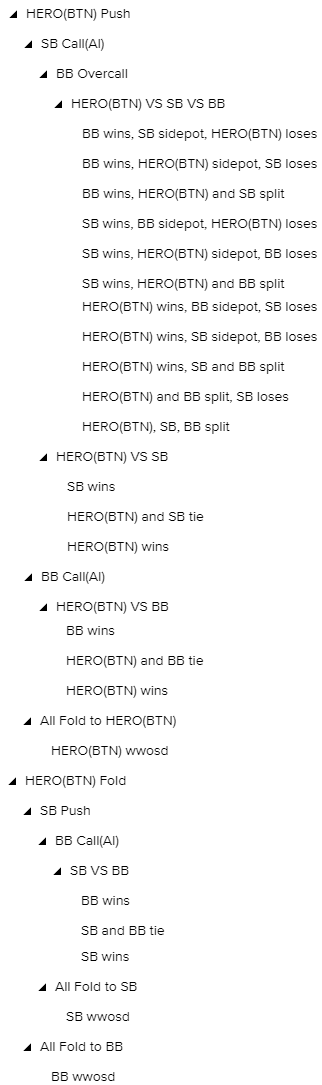1. After that, we need to calculate the probability of each outcome.

We'll need to pay attention to the card removal effect, apply combinatorial formulas, and use the concept of conditional probability. For example, to calculate the probability of a BB overcall, we need to multiply the probability of the hero pushing, the SB call range in the event of a push, and the BB overcall range for an SB call.

Let's look at a simple example of how the probability of the SB calling with a 3.8% call range (TT+,AQs+,AKo) is calculated.

The TT+,AQs+,AKo range includes:

5 pairs + 1 offsuit unpaired hand + 2 suited unpaired hands = 5 × 6 possible combinations of each paired hand + 1 × 12 possible combinations of each offsuit unpaired hand + 2 × 4 possible combinations of each suited unpaired hand = 30 + 12 + 8 = 50 combinations.

There are a total of 169 hands on the pushfold table.

13 pairs + 78 offsuit unpaired hands + 78 suited unpaired hands = 13 × 6 possible combinations of each paired hand + 78 × 12 possible combinations of each offsuit unpaired hand + 78 × 4 possible combinations of each suited unpaired hand = 78 + 936 + 312 = 1,326 combinations.

If we were to calculate the probability of the SB calling with a clean call range without accounting for the card removal effect, then, logically, the probability of getting the top 3.8% of hands would be approximately 3.8%:

(50 / 1,326) × 100% = 3.77%

But our AA are blocking some of the hands from the SB call range, and we have to account for the card removal effect. In this case, the calculation would look like this:

TT+,AQs+,AKo = 4 × 6 possible combinations of each paired non-AA hand + 1 possible AA combination + 1 × 6 possible combinations of each offsuit unpaired hand + 2 × 2 possible combinations of each suited unpaired hand = 24 + 1 + 6 + 4 = 35 combinations.

Since the deck no longer contains two hero cards, there are only 1,225 possible combinations left in the pushfold table.

As a result, the probability of an SB call with a 3.8% range = (35 / 1,225) × 100% = 2.8571% ≈ 2.86%.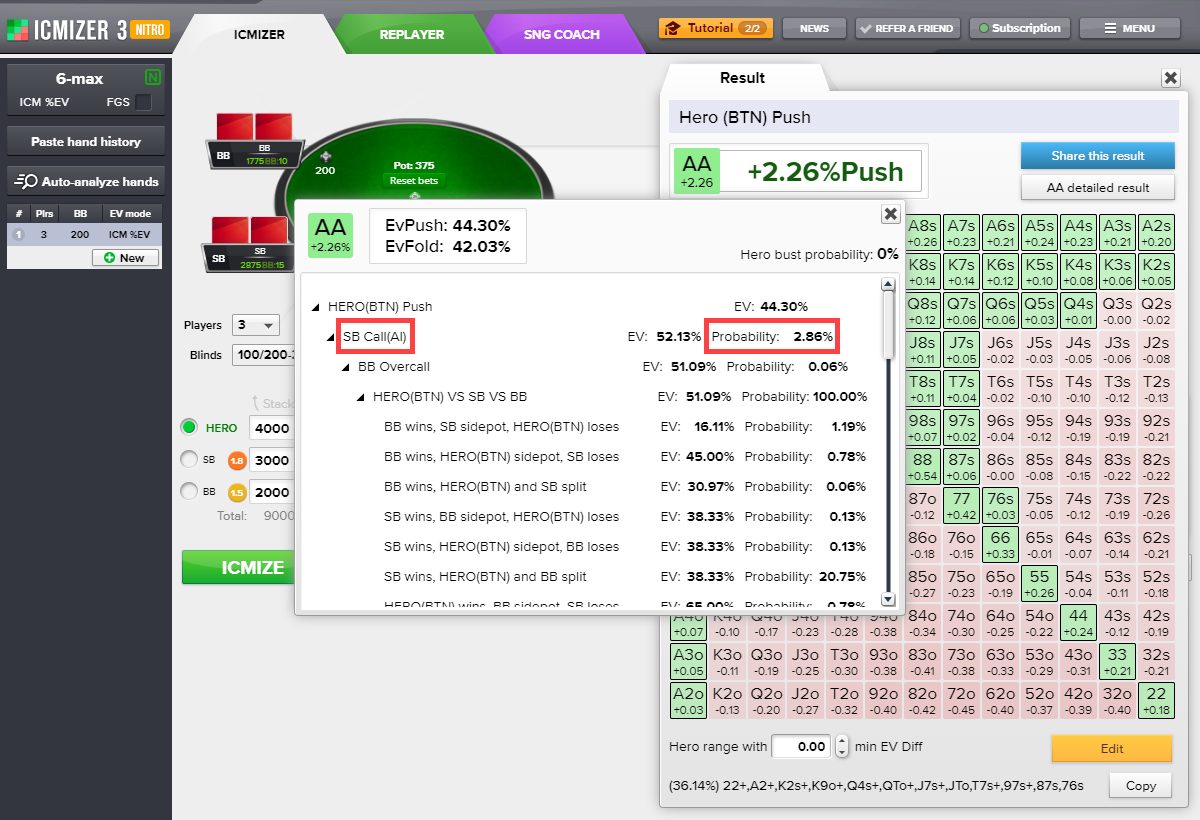In two-player pots, there are three possible outcomes (the first one wins, the second one wins, split). In three-player pots, there are 13 different outcomes. We calculate the probability of each one.

1. After we calculate the probability of each outcome, we need to calculate the change in the hero's tournament share for each one. Since we already know the payout structure and players' stacks for a specific outcome, we'll get the EV for each outcome.

Let's suppose that the BTN has enough reason to think the BB will go all-in with the following range: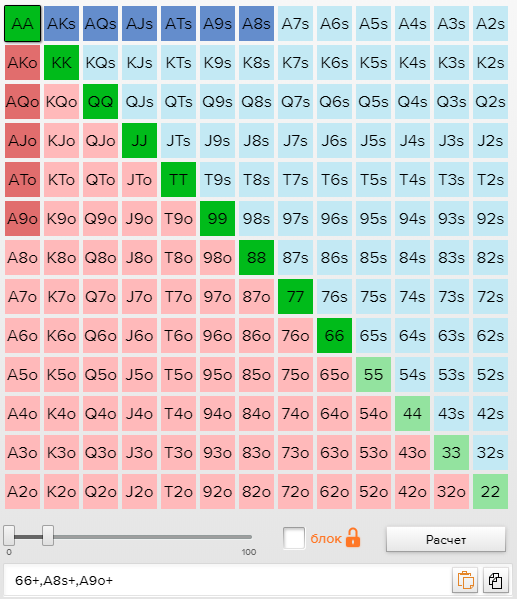[66+,A8s+,A9o+]

Using a simple equity calculator, we see that with an AA:

The BTN has:

An 84.46% chance of beating a BB call
A 1.85% chance of drawing
A 13.69% probability of losing.

We can see this in the hand detailed result: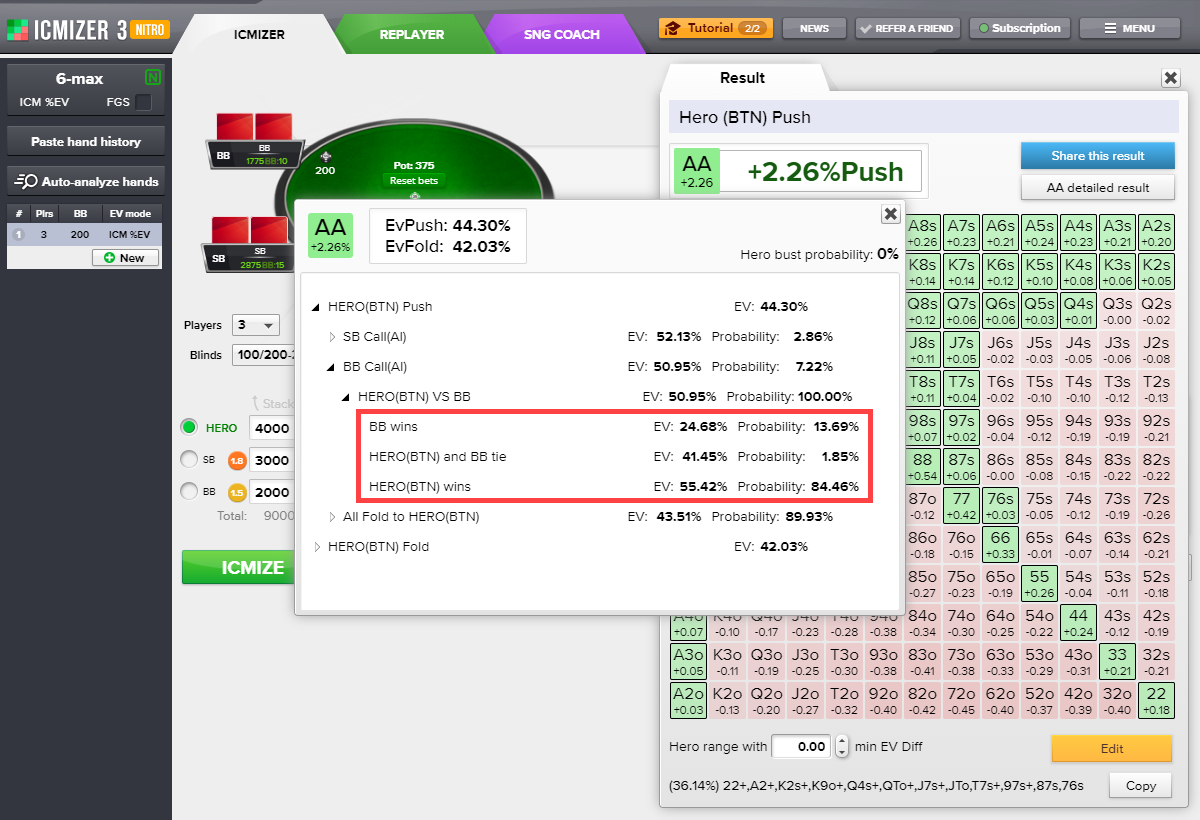84.46% of the time, the resulting stacks will look like this:

BTN: 6,125
SB: 2,875
BB: 0

1.85% of the time, when BTN and BB draw and split the SB bet, the resulting stacks will be:

BTN: 4,063
SB: 2,875
BB: 2,062

13.69% of the time, the BTN loses when going all-in:

BTN: 1,975
SB: 2,875
BB: 4,150

Set the values of all total stacks in the ICM Calculator to calculate the ICM EV for each result, multiply their EVs accordingly with the probabilities that they'll occur, and add those numbers. That gives us the total ICM EV when calling an all-in.

https://www.icmpoker.com/icmcalculator/#RXPQ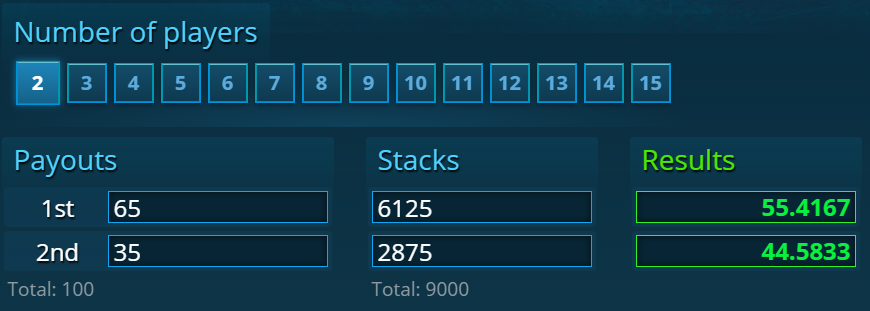https://www.icmpoker.com/icmcalculator/#pTTh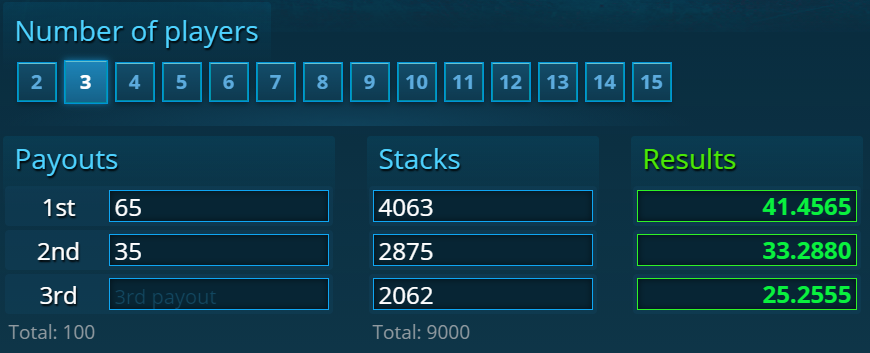https://www.icmpoker.com/icmcalculator/#JHps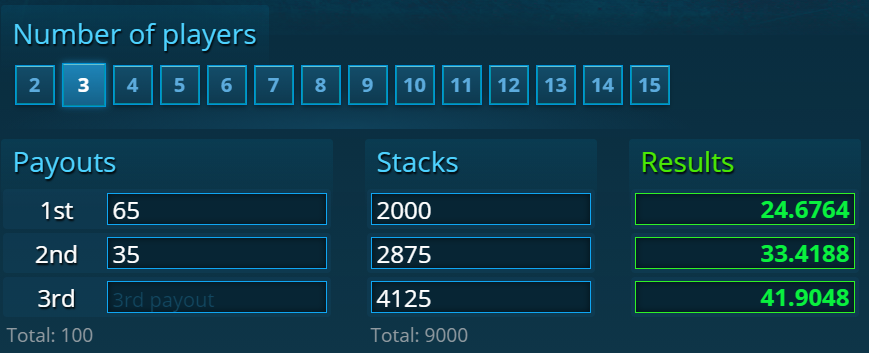We end up with:

 EV (% of the prize pool) Probability Win 55.42 84.46% Draw 41.46 1.85% Loss 24.68 13.69%

We multiply the EV of each final stack by the probability and add these numbers to get the total EV of an all-in with AA in this situation. The formula for the calculation looks like this:

Hand win% × ICM EV win + Hand tie% × ICM EV tie + Hand lose% × ICM EV lose

(0.5542 × 0.8446) + (0.4146 × 0.0185) + (0.2468 × 0.1369) = 0.5095 = 50.95 EV

ICMIZER agrees with us: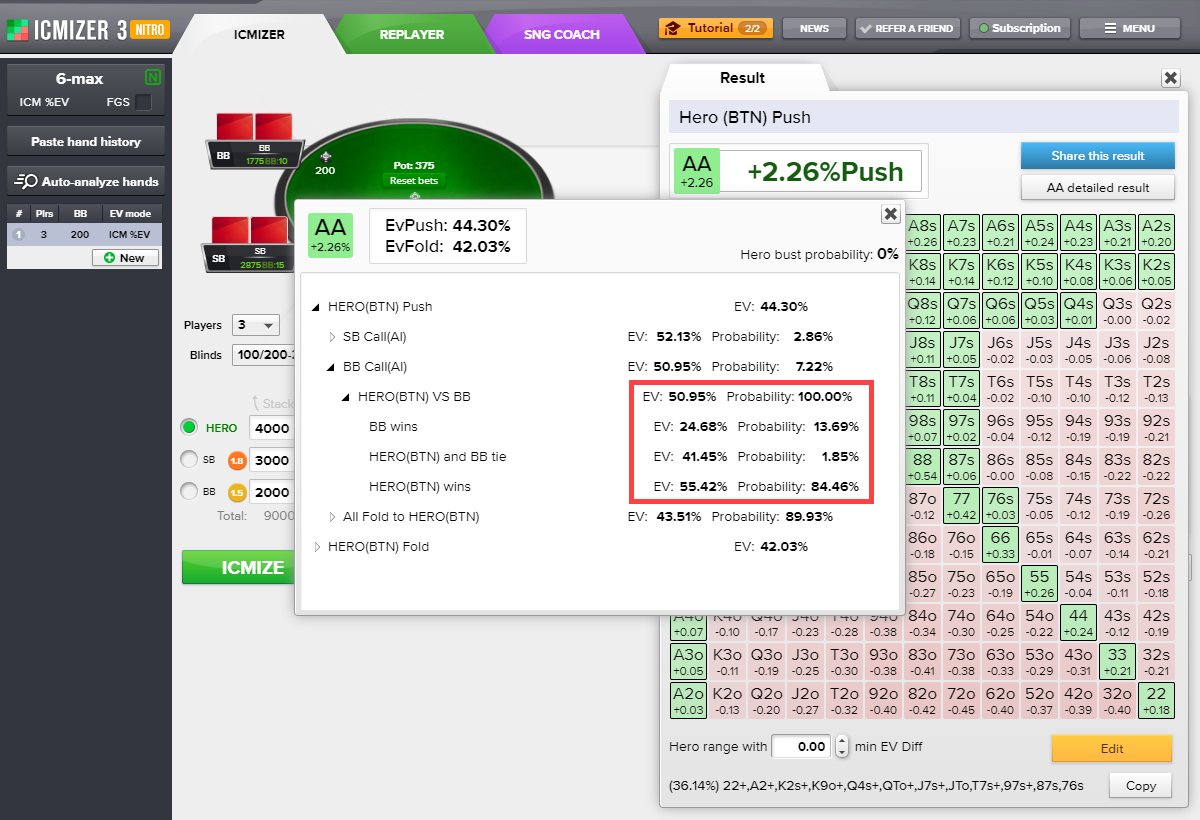Now that we know the EV of calling an all-in with AA, we need to know the EV of a fold, the expected value of the tournament share in the event of a fold. Without knowing the EV for a fold, we wouldn't have a value we could compare to the EV of calling an all-in.

If the BTN folds, he loses 25 chips for the ante, SB loses 125 chips, BB takes the pot, and the stacks look like this:

BTN: 3,975
SB: 2,875
BB: 2,150

In this case, this formation gives the BTN an EvFold of 40.82%.

https://www.icmpoker.com/icmcalculator/#rXYE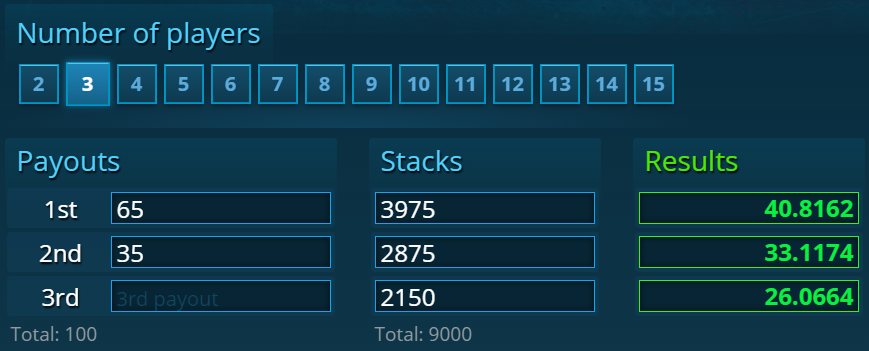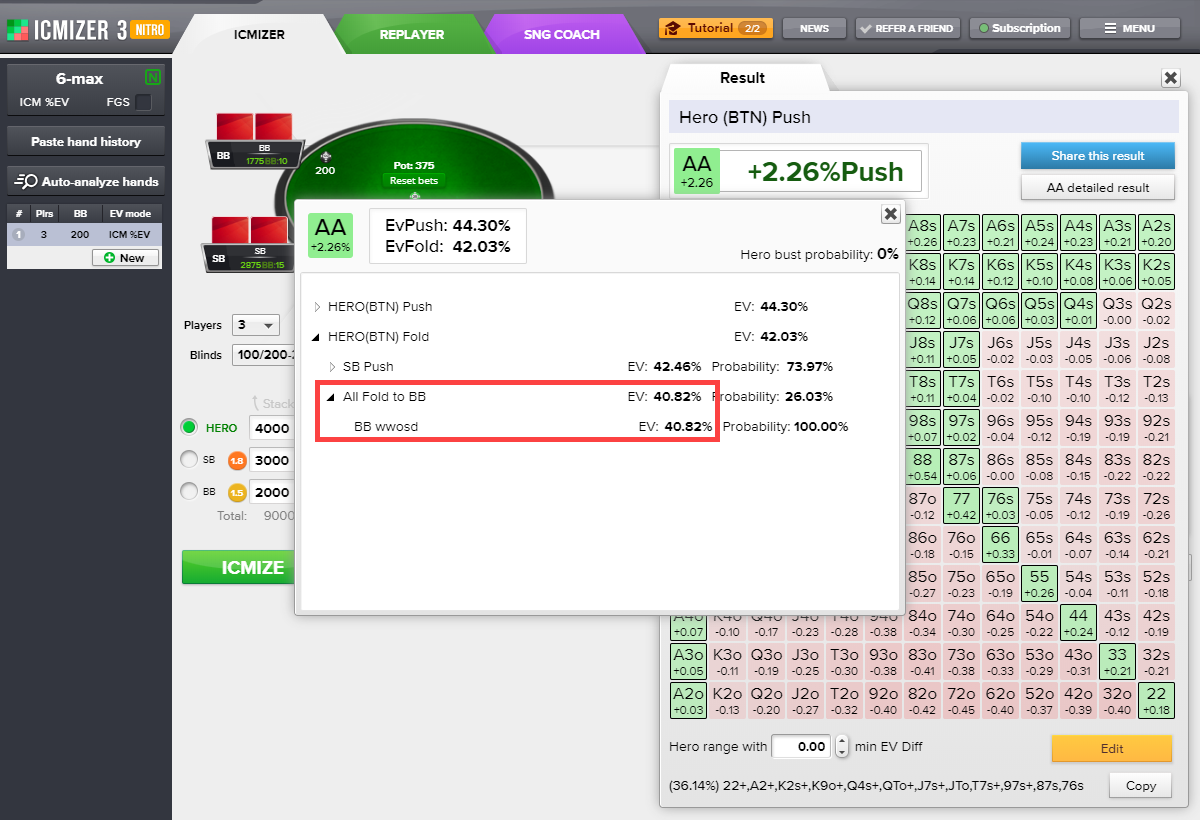When we compare this to the EV of a push (50.95%), we see that pushing against the BB is more profitable by (50.95 - 40.82) = 10.13% of the prize pool than folding.

EvPush BB Overcall and EvPush vs SB are calculated in the same way: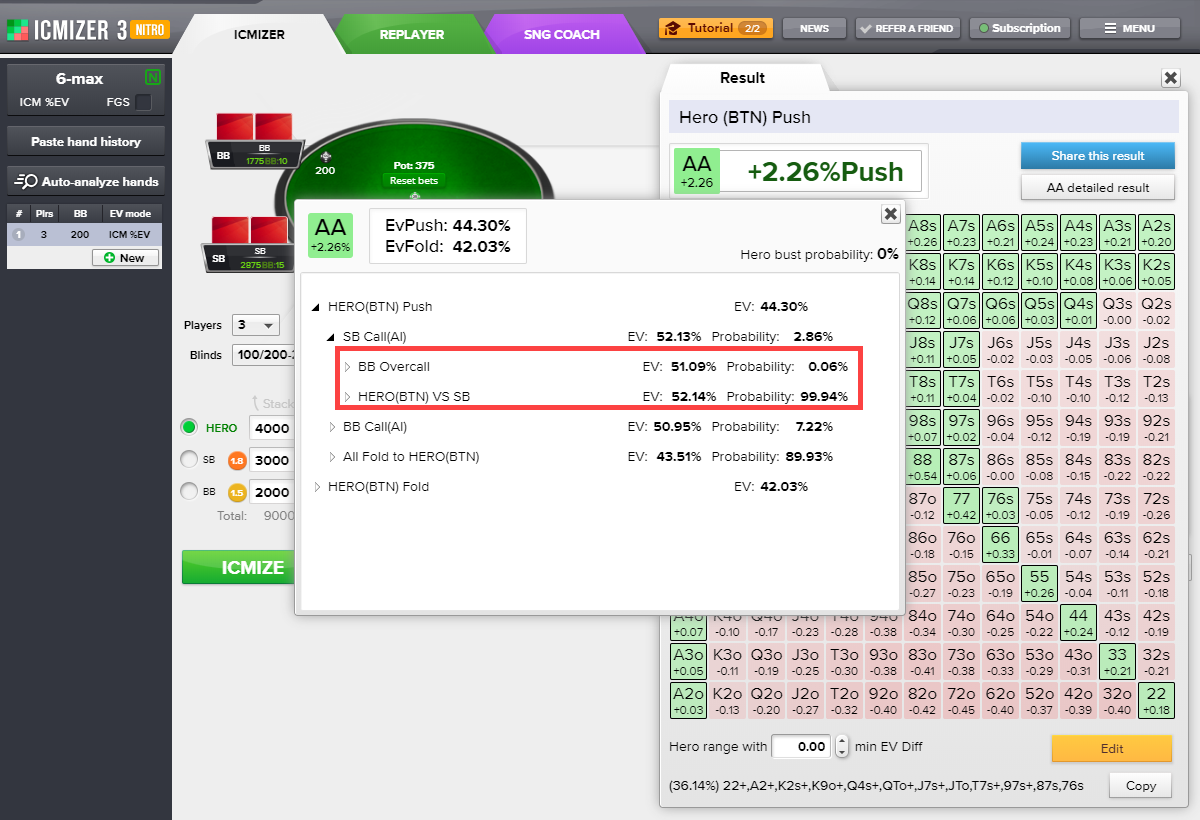To calculate the total EV of a push, we need to find the sum of the probabilities of outcomes for the EVs corresponding to them. In other words, the EV of a push is the weighted sum of the EVs of each potential outcome. The weight is the actual probability of each outcome. Therefore, the lower the probability of an outcome is, the less weight it has.

As such, we have the hero's EvPush against the BB calling (50.95%), which we calculated above, and the hero's EvPush against the SB calling:

(BB Overcall EV × BB Overcall Probability) + (Hero vs SB EV × Hero vs SB EV Probability) = (51.09 × 0.0006) + (52.14 × 0.9994) = 0.03 + 52.10 = 52.13%

We also need to find the hero's EvPush if everyone folds on his push. It's easy to calculate the probability of such an outcome:

All Fold to Hero Probability = 100 – (SB Call Probability + BB Call Probability) = 100 – 2.8571 (which we calculated above) – 7.22 ≈ 89.93%

Now let's calculate the hero's EvPush when everyone folds on his push. The stacks will look like this:

BTN: 4,350
SB: 2,875
BB: 1,775

In this case, this formation gives the BTN an EV of 43.51%.

https://www.icmpoker.com/icmcalculator/#oRNJ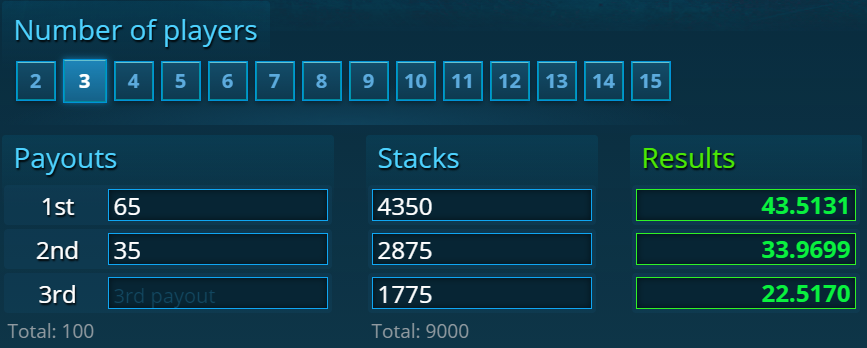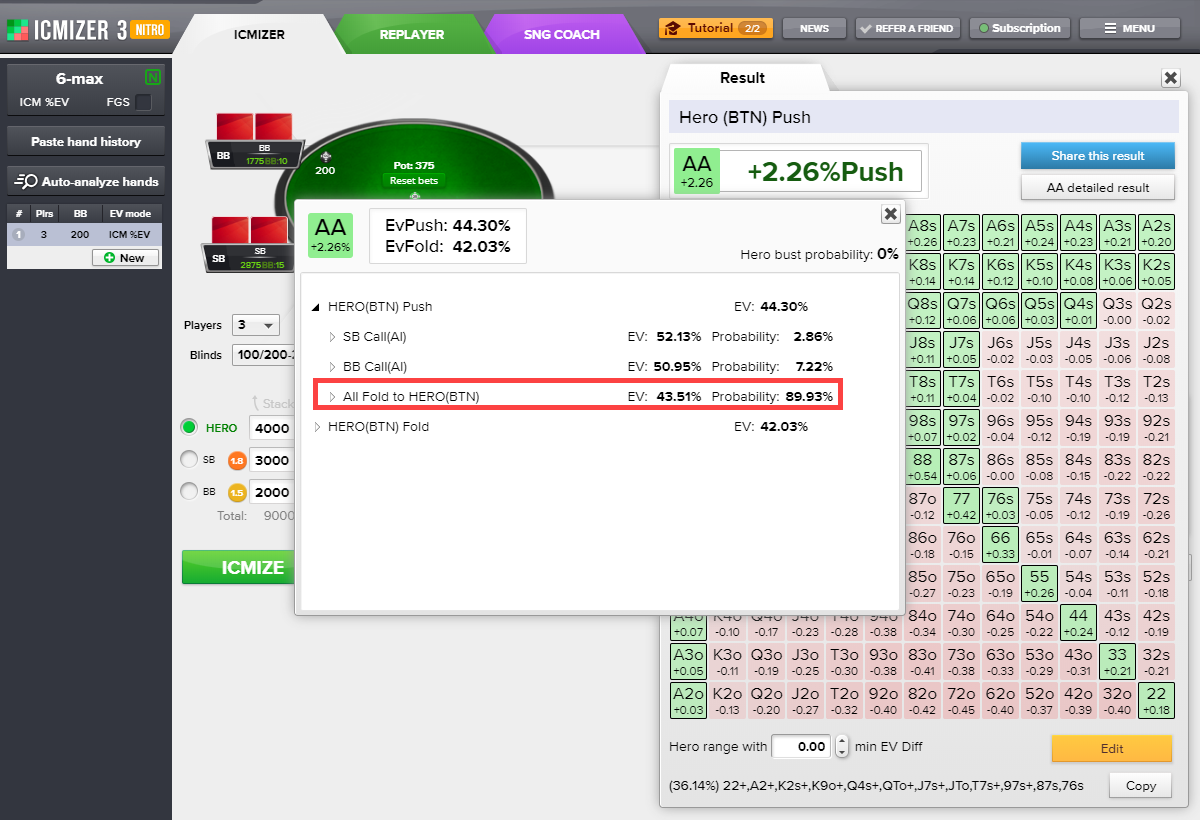Total EvPush = (SB Call EV × SB Call Probability) + (BB Call EV × BB Call Probability) + (All Fold to Hero EV × All Fold to Hero Probability) = (52.13 × 0.0286) + (50.95 × 0.0722) + (43.51 × 0.8993) = 1.4909 + 3.6786 + 39.1285 = 44.30%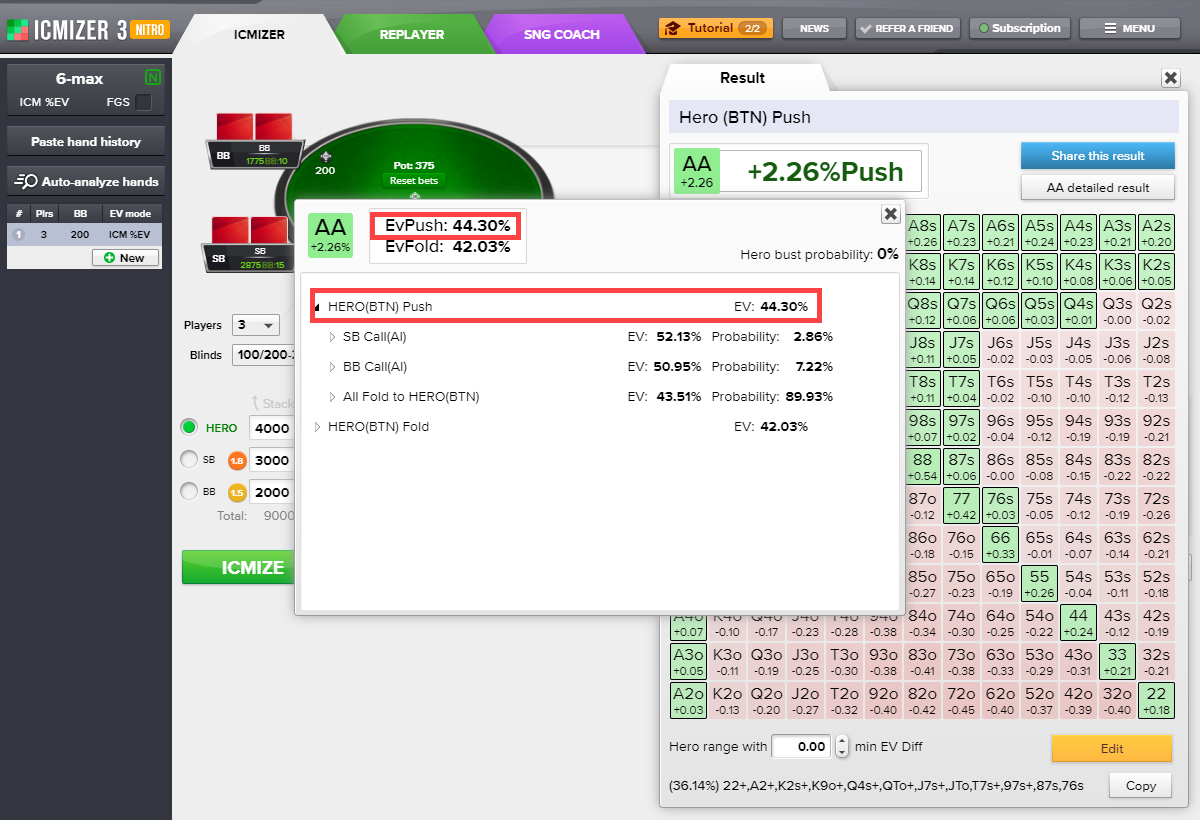Now that we know the total EV of the hero's push with AA, we need to know the total EV of folding.

Total EvFold = (SB Push EV × SB Push Probability) + (All Fold to BB EV × All Fold to BB Probability)

We calculated the EV of the hero folding against the BB above; it's 40.82%.

We'll skip how to calculate SB Push EV and Probability, and All Fold to BB Probability since they're calculated the same way as the process described above. Instead, we'll move straight to calculating the total EvFold:

(42.46 × 0.7397) + (40.82 × 0.2603) = 42.03%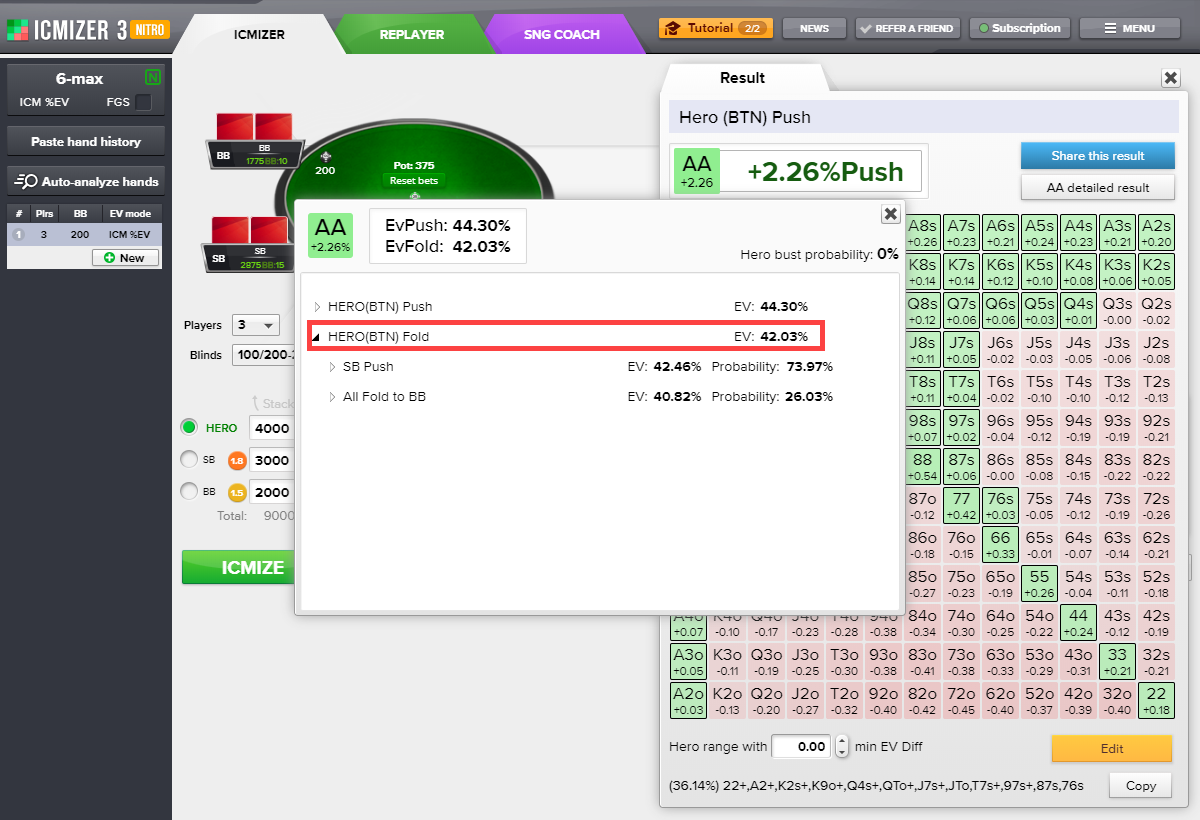When we compare this to the EV of a push (44.30%), we see that pushing is more profitable by (44.30 - 42.03) = 2.26% of the prize pool than folding.

For an inexperienced player, 2.26% may not seem like a lot, but it's actually enormous equity that yields more money per game decision than the best single-table SNG tournament players can get on average for the entire game.